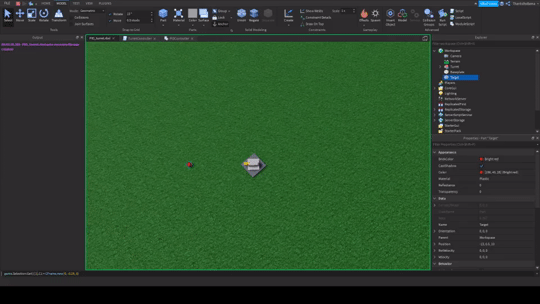# How do I apply PID to CFrame lerping?

So I saw the tutorial based on PID and I was amazed at how you can apply it to non-physics based objects like a GUI.

I think it would be really cool if I could apply it to CFrame lerping in order to obtain physics like results specifically for moving a turret so it oscillates and feels more realistic and doesn’t always 100% match the target.

Here’s the resource I’m wanting to apply PID to, you can download it experiment if you want.

Here is the code bit related to lerping.

``````local adjustedLerpAlpha
if step and self.ConstantSpeed then
local angularDistance = VectorUtil.AngleBetween(currentRotation.LookVector,goalRotationCFrame.LookVector)
local estimatedTime = self.AngularSpeed/angularDistance
elseif step then
end

local newRotationCF = currentRotation:lerp(goalRotationCFrame, adjustedLerpAlpha or self.LerpAlpha)

self.JointMotor6D.C0 = CFrame.new(originalC0Position)*newRotationCF
``````

So yeah given two CFrames the current and the goal how can I adjust the lerp alpha in a RunService connection to apply a “Force” through PID.

1 Like

NVM, it’s not possible with the current setup because of how lerping towards the goal works where it’ll only be in a value of 0-1 so it’s hard to find the directionality of the angular velocity we want to control with PID and apply to the current rotation.

I’ll take a break from this problem for now, I believe the solution is to align the CFrame axis like how the AlignOrientation bodymover works by getting the rotation between two vectors of the axis of the attachments.

This is a really cool idea! I might give it a shot and add it as an example use case. If I do I’ll comment hereEdit: it works quite well, but it definitely needs a PD controller to deal with the oscillations. But with a PD controller it’s super cool! I’ll probably use it as the main example when I cover D control.

1 Like

Found the solution, using everyone’s favorite axis angle rotation representationAll CFrames no AlignOrientationCode
``````local RunService = game:GetService("RunService")

local part = script.Parent
local target = workspace.gun.Target --random part in a model

function p_controller(kP, set_point, process_value)
local e = set_point - process_value
local CO = kP * e
return CO
end

local partAngularVelocity = Vector3.new(0,0,0)
local goal = Vector3.new()

RunService.Heartbeat:Connect(function(dt)
local partCF = part.CFrame
local targetCF = target.CFrame
local differenceCF = partCF:ToObjectSpace(targetCF)
local axis, angle = differenceCF:ToAxisAngle()
--obtain rotation difference in form of torque
--axis is well yeah rotation axis
local test = axis*angle
--go towards goal rotation of zero difference
local outputAcceleration = p_controller(0.1,goal,test)

--add torque difference into the parts current velocity
partAngularVelocity += -outputAcceleration*dt

--Convert current angular velocity into CFrame representation
local newaxis = partAngularVelocity.Unit
local newangle = partAngularVelocity.Magnitude

local stuff
-- if there is a rotation or else it's a .Unit Nil error
if newaxis == newaxis then
stuff = CFrame.fromAxisAngle(newaxis,newangle)
else
stuff = CFrame.new()
end
part.CFrame *= stuff-stuff.Position

end)
``````

Yeah, it definitely needs something to stop the oscillations. but wow it does look smooth as if it has physics enabled. Seems like D control will be required looking forward to your follow up tutorial!

1 Like

Nice!Here’s what I’ve come up with, it only rotates on the yaw axis, I was thinking it’d be interesting to have yaw and pitch be different “hinges” / Motor6Ds:TurretController
``````local RunS = game:GetService("RunService")
local PIDController = require(game.ServerStorage.PIDController)
PIDController.update_time_on(RunS.Heartbeat)

local turret = script.Parent
local turretBase = turret.Base
local yawBase = turret.YawAssembly.YawBase
local yawMotor = turret.Base.YawMotor6D

local target = game.Workspace.Target

local yaw_velocity = 0
local yaw_controller = PIDController.new_PID_controller(10, 0, 3, .1)

RunS.Heartbeat:Wait()

RunS.Heartbeat:Connect(function(dt)
local object_horizontal_offset = yawBase.CFrame:PointToObjectSpace(target.Position)
local object_yaw_angle = math.atan2(-object_horizontal_offset.X, -object_horizontal_offset.Z)

local yaw_force = yaw_controller(0, object_yaw_angle)
yaw_velocity += yaw_force * dt

yawMotor.C1 *= CFrame.Angles(0, yaw_velocity * dt, 0)
end)
``````
PIDController Module

local t = tick()

local update_c

function update_time()
t = tick()
end

function update_time_on(event)
if update_c then
update_c:Disconnect()
end

``````update_c = event:Connect(update_time)

return update_c
``````

end

function new_P_controller(kP)
return function(SP, PV)
local e = SP - PV
return kP * e
end
end

function new_I_controller(kI, example_value)
local prev_t = t
local sum_e = 0 * (example_value or 0)

``````return function(SP, PV)
local dt = t - prev_t
prev_t = t

local e = SP - PV

sum_e += e * dt
return kI * sum_e
end
``````

end

function new_D_controller(kD, initial_error)
local prev_t = t
local prev_e = initial_error

``````return function(SP, PV)
local dt = t - prev_t
prev_t = t

local e = PV - SP
local de = e - prev_e

local de_dt = de / dt

prev_e = e
return kD * -de_dt
end
``````

end

function new_PID_controller(kP, kI, kD, initial_error)
local P_controller = new_P_controller(kP)
local I_controller = new_I_controller(kI, initial_error)
local D_controller = new_D_controller(kD, initial_error)

``````return function(SP, PV)
return P_controller(SP, PV)
+ I_controller(SP, PV)
+ D_controller(SP, PV)
end
``````

end

local Module = {
update_time = update_time,
update_time_on = update_time_on,
new_P_controller = new_P_controller,
new_I_controller = new_I_controller,
new_D_controller = new_D_controller,
new_PID_controller = new_PID_controller,
}
return Module

I haven’t put the PID module on the tutorial thing because I’m not quite happy with it yet, I want to polish it a bit more before releasing it properly.

EDIT: Added the pitch Motor6D w/ a separate controller, here’s a video:

... and here's the updated TurretController
``````
local RunS = game:GetService("RunService")
local PIDController = require(game.ServerStorage.PIDController)
PIDController.update_time_on(RunS.Heartbeat)

local turret = script.Parent
local yawBase = turret.YawAssembly.YawBase
local pitchBase = turret.YawAssembly.PitchAssembly.PitchBase
local yawMotor = turret.YawBase.YawMotor6D
local pitchMotor = turret.YawAssembly.PitchBase.PitchMotor6D

local target = game.Workspace.Target

local yaw_velocity = 0
local pitch_velocity = 0
local yaw_controller = PIDController.new_PID_controller(8, 0, 5, .1)
local pitch_controller = PIDController.new_PID_controller(10, 0, 2, .1)

RunS.Heartbeat:Wait()

RunS.Heartbeat:Connect(function(dt)
local object_yaw_offset = yawBase.CFrame:PointToObjectSpace(target.Position)
local object_yaw_angle = math.atan2(-object_yaw_offset.X, -object_yaw_offset.Z)
local yaw_force = yaw_controller(0, object_yaw_angle)
yaw_velocity += yaw_force * dt
yawMotor.C1 *= CFrame.Angles(0, yaw_velocity * dt, 0)

local object_pitch_offset = pitchBase.CFrame:PointToObjectSpace(target.Position)
local object_pitch_angle = math.atan2(object_pitch_offset.Y, -object_pitch_offset.Z)
local pitch_force = pitch_controller(0, object_pitch_angle)
pitch_velocity += pitch_force * dt
pitchMotor.C1 *= CFrame.Angles(pitch_velocity * dt, 0, 0)
end)

``````
4 Likes